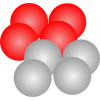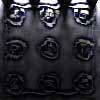#### You may also like### Fixing the Odds

You have two bags, four red balls and four white balls. You must put all the balls in the bags although you are allowed to have one bag empty. How should you distribute the balls between the two bags so as to make the probability of choosing a red ball as small as possible and what will the probability be in that case?### Scratch Cards

To win on a scratch card you have to uncover three numbers that add up to more than fifteen. What is the probability of winning a prize?### Escalator

At Holborn underground station there is a very long escalator. Two people are in a hurry and so climb the escalator as it is moving upwards, thus adding their speed to that of the moving steps. ... How many steps are there on the escalator?

# Concrete Calculation

##### Age 14 to 16Challenge Level

Several solutions arrived this month but some of you fell into the trap of assuming that the sides of the frustrum would converge to a point but they do not so the solution needed you do dissect the mound in the middle as suggested here by Michael Levitin of St. Margaret's Episcopal School San Juan Capistrano, California, USA. I like your advice to the builder Michael, how about a spreadhsheet?

The answer is 23.154 cubic meters.

The solution:

First we figure out the volume of the whole rectangular prism. Then we figure out the volume of the frustrum of the pyramid. Then we simply subtract the volume of the frustrum from the volume of the rectangular prism.

The volume of the rectangular prism is the area of the base times the height. So that is 6 x 5 x 12 = 36 cubic meters.

To calculate volume of the frustrum:

Put the frustum on a cutting board with the bigger base down. Take a huge sharp knife and make four straight cuts along the edges of the top square. Then we will have nine pieces.

And they will be:

One rectangular prism with base sides of 3.8 m by 2.8 m and with height of 0.9 m. Then the volume will be 3.8 x 2.8 x 0.9 = 9.576 cubic meters.

We will also have four triangular prisms from the sides of frustrum that we will put one on top of the other to form a triangular prism with the right triangle base with legs of 0.5 m and 0.9 m and area of (0.5 x 0.9)/2 = 0.225 square meters. The height of the triangular prism is the perimeter of the frustrum top base and is 2 x (3.8 + 2.8) = 13.2 m. So the volume of the triangular prism is the area of the base times height and equal to 0.225 x 13.2 = 2.97 cubic meters.

We will combine four corners of the frustrum and make a pyramid with a square base with sides of 1 m and a height of 0.9 m. Then the area of the base is 1 square meter and the volume is 1 x 0.9 / 3 = 0.3 cubic meters.

Then we find the volume of the frustrum as the sum of the volume of the figures. 9.576 + 2.97 + 0.3 = 12.846 cubic meters. Then we subtract the volume of the frustrum from the volume of the rectangular prism 36 - 12.846 = 23.154 cubic meters.

Suggestion to the builders: Either do what we have done or make a computer program that does it.